# R S Aggarwal Solutions for Class 10 Maths Chapter 4 Quadratic Equations Exercise 4B

Get R S Aggarwal Solutions for Class 10 Maths Chapter 4 Quadratic Equations exercise 4B here. This exercise is based on solving quadratic equations by completing the square method. Download free pdf by clicking on the link given below and start practicing the R S Aggarwal Solutions for Class 10 to sharpen your skills.

## Download PDF of R S Aggarwal Solutions for Class 10 Maths Chapter 4 Quadratic Equations Exercise 4B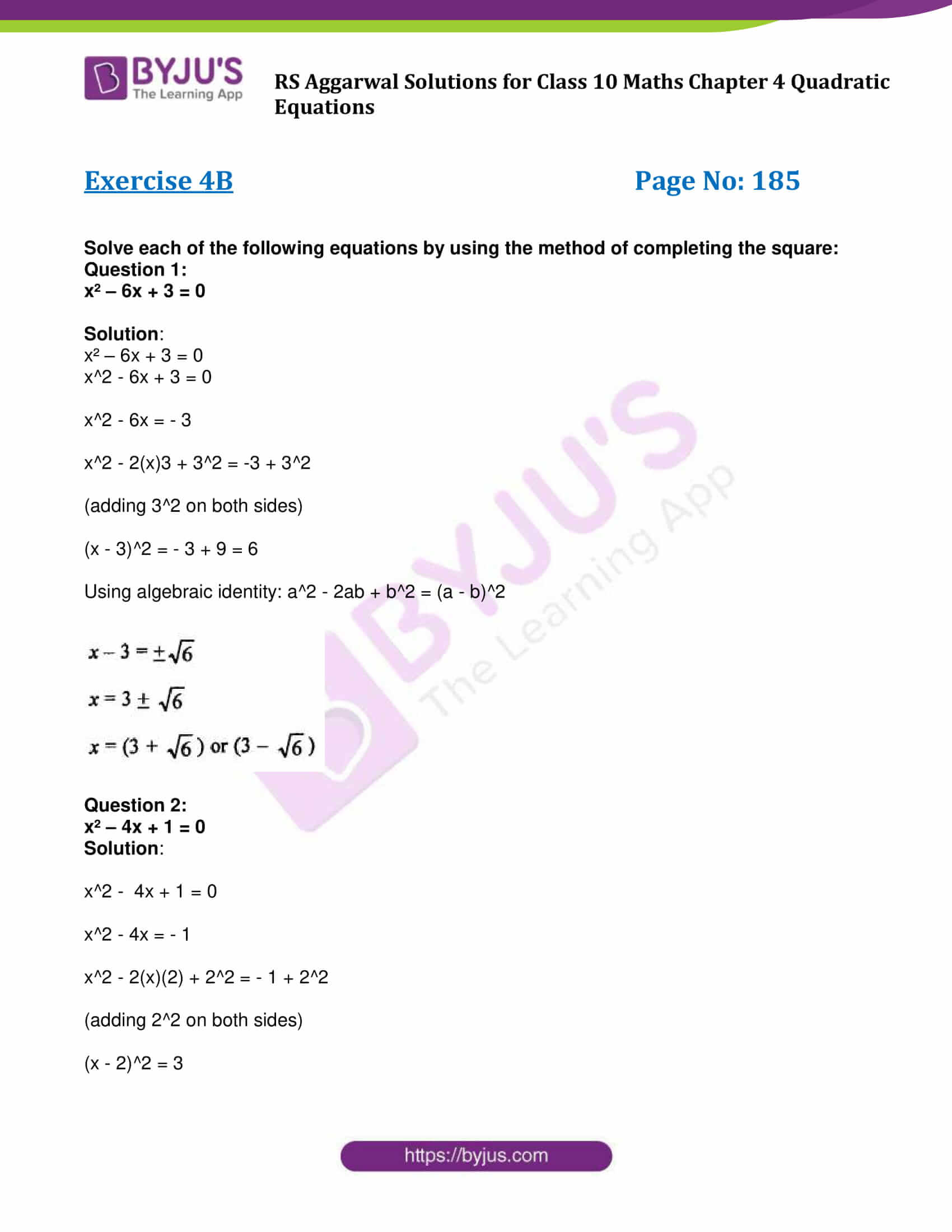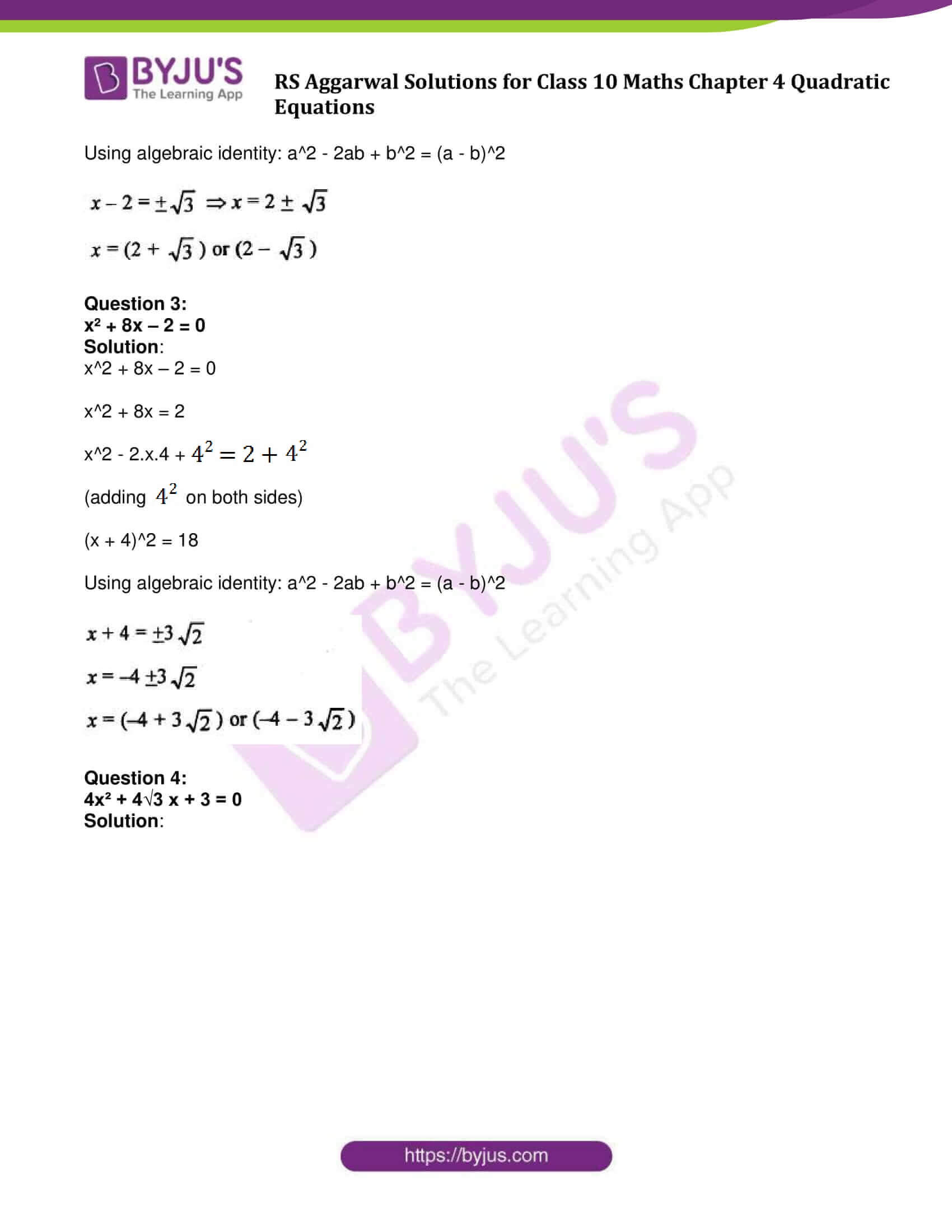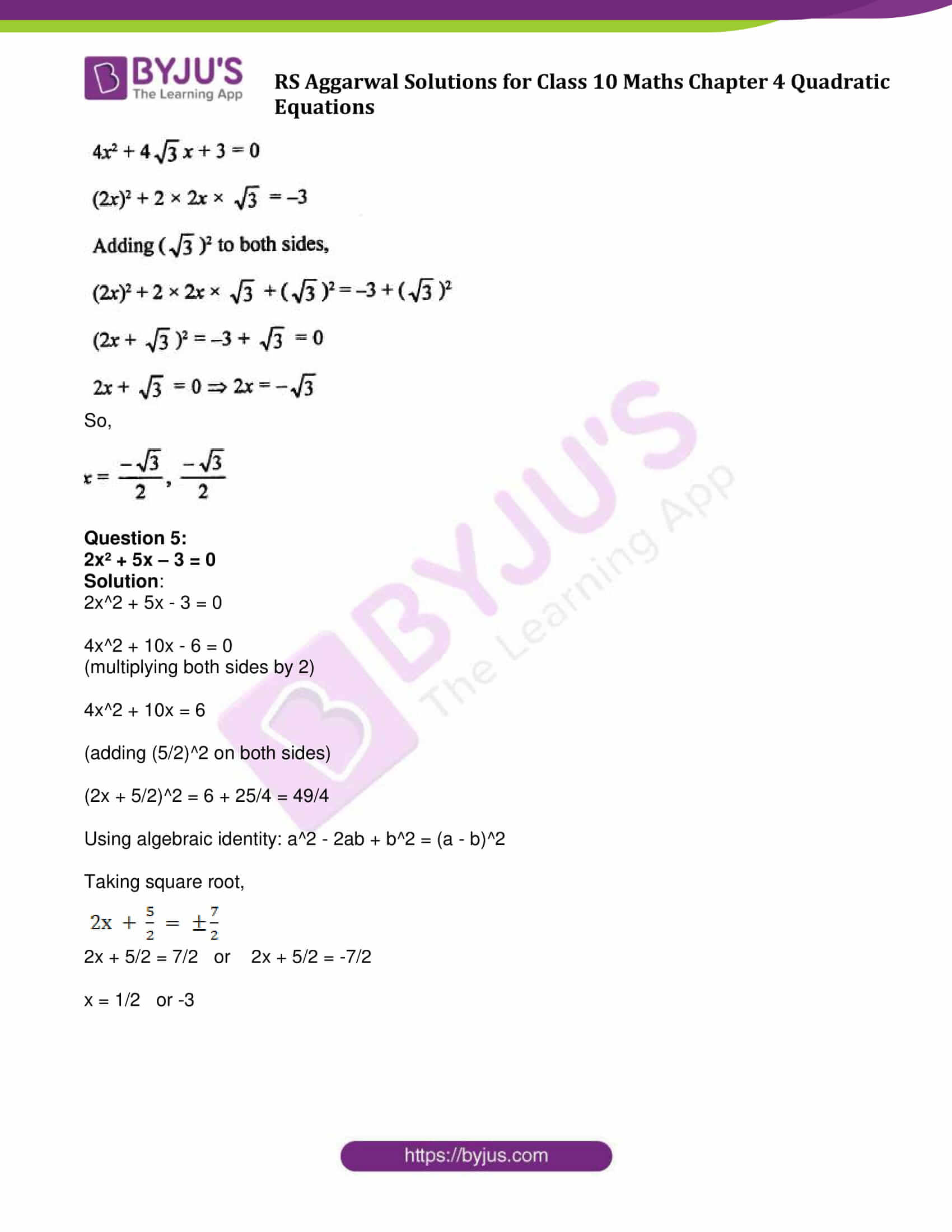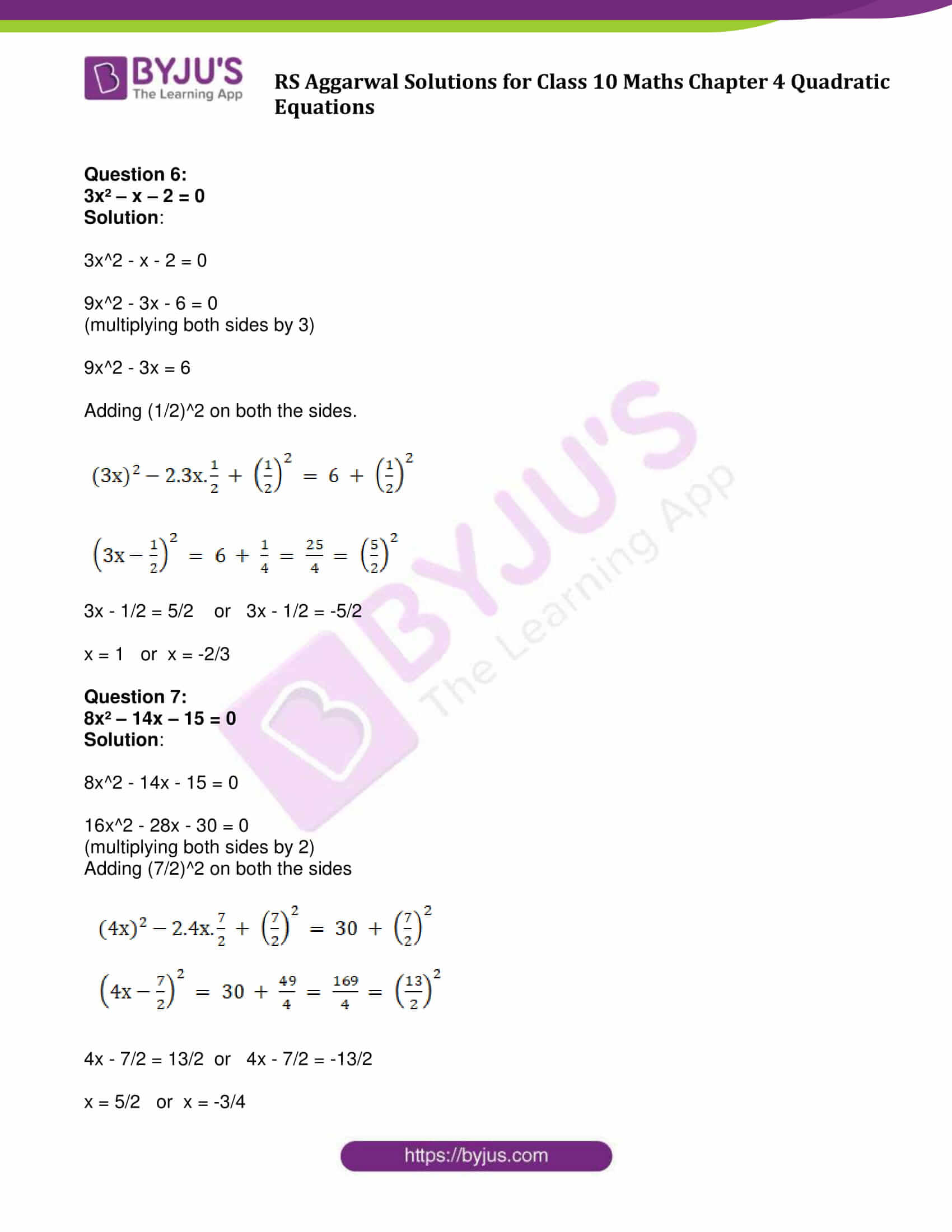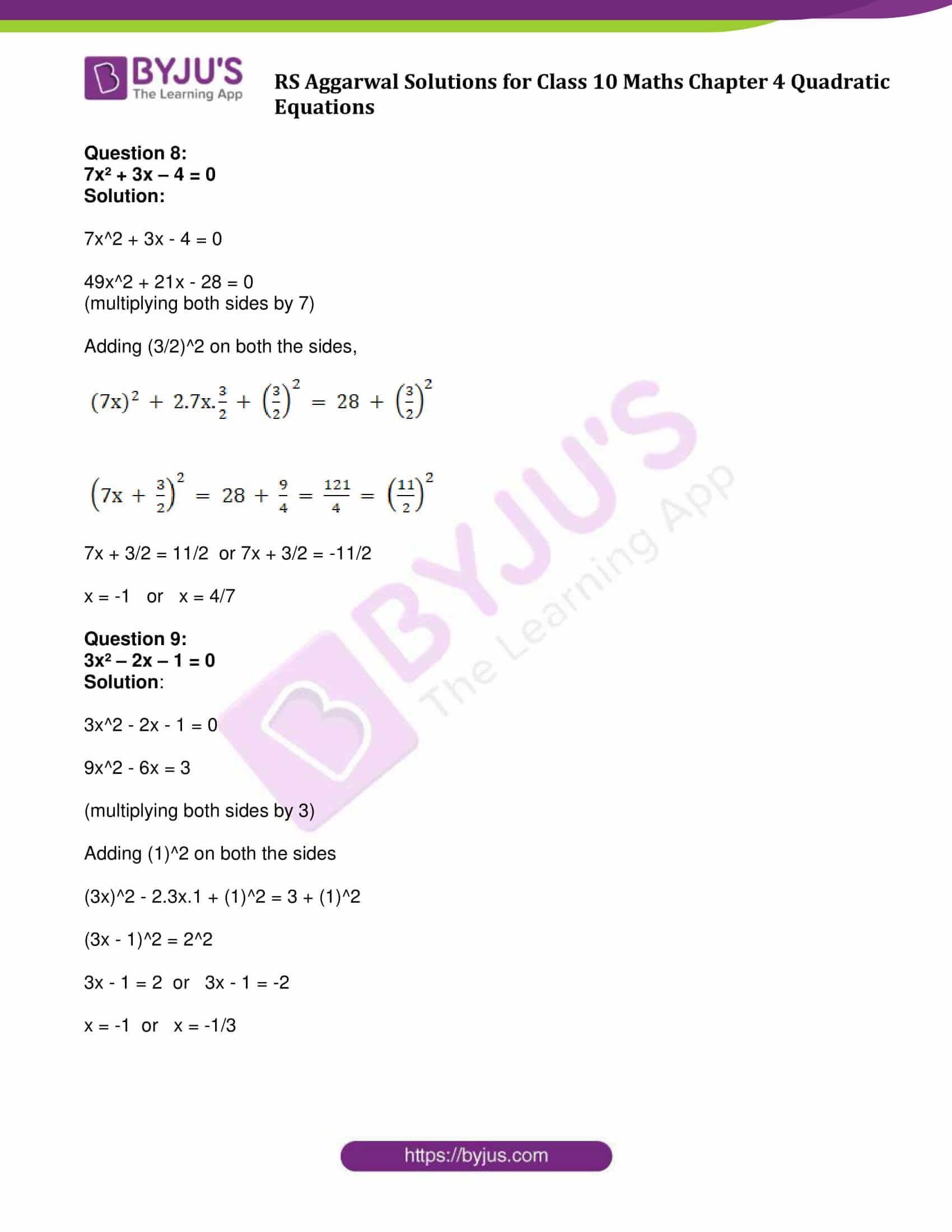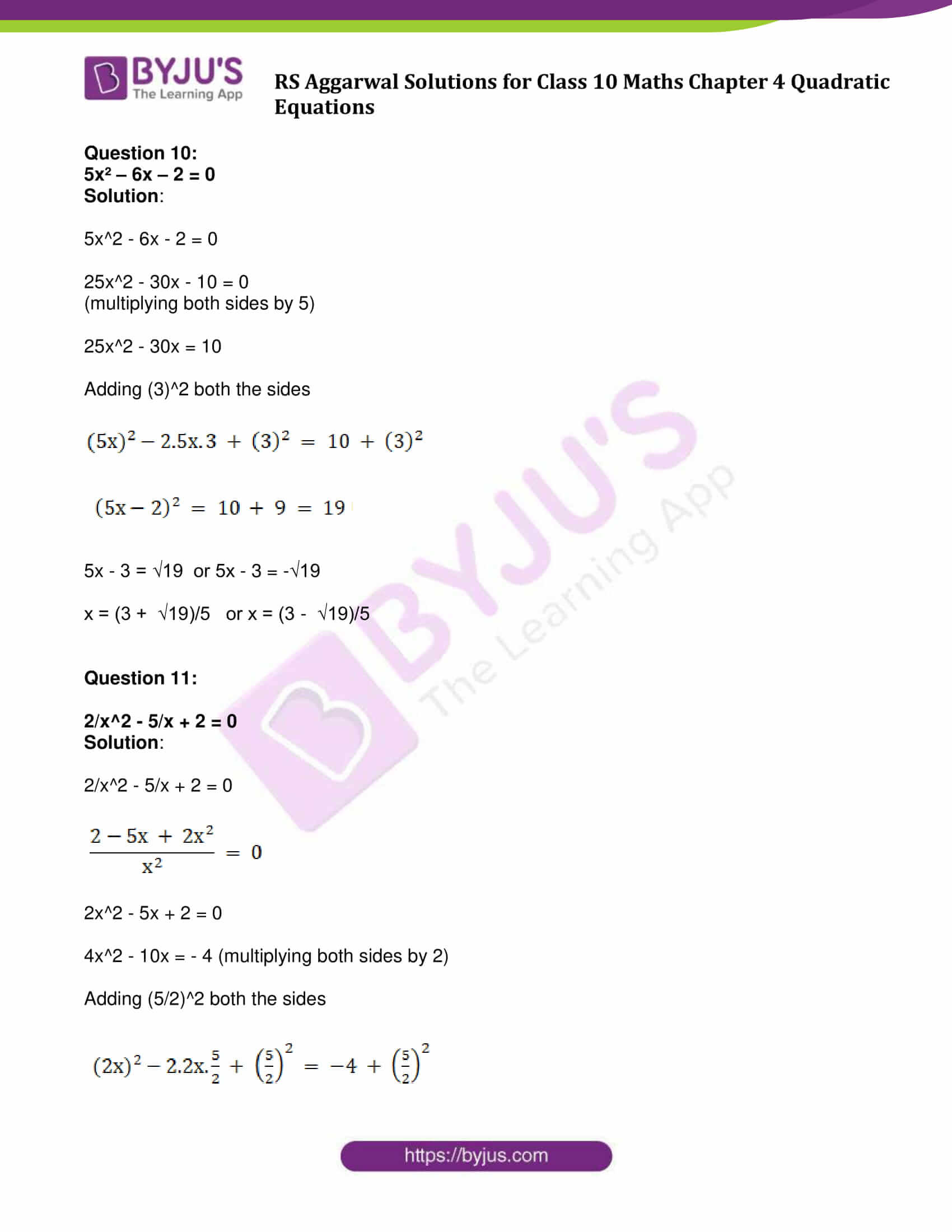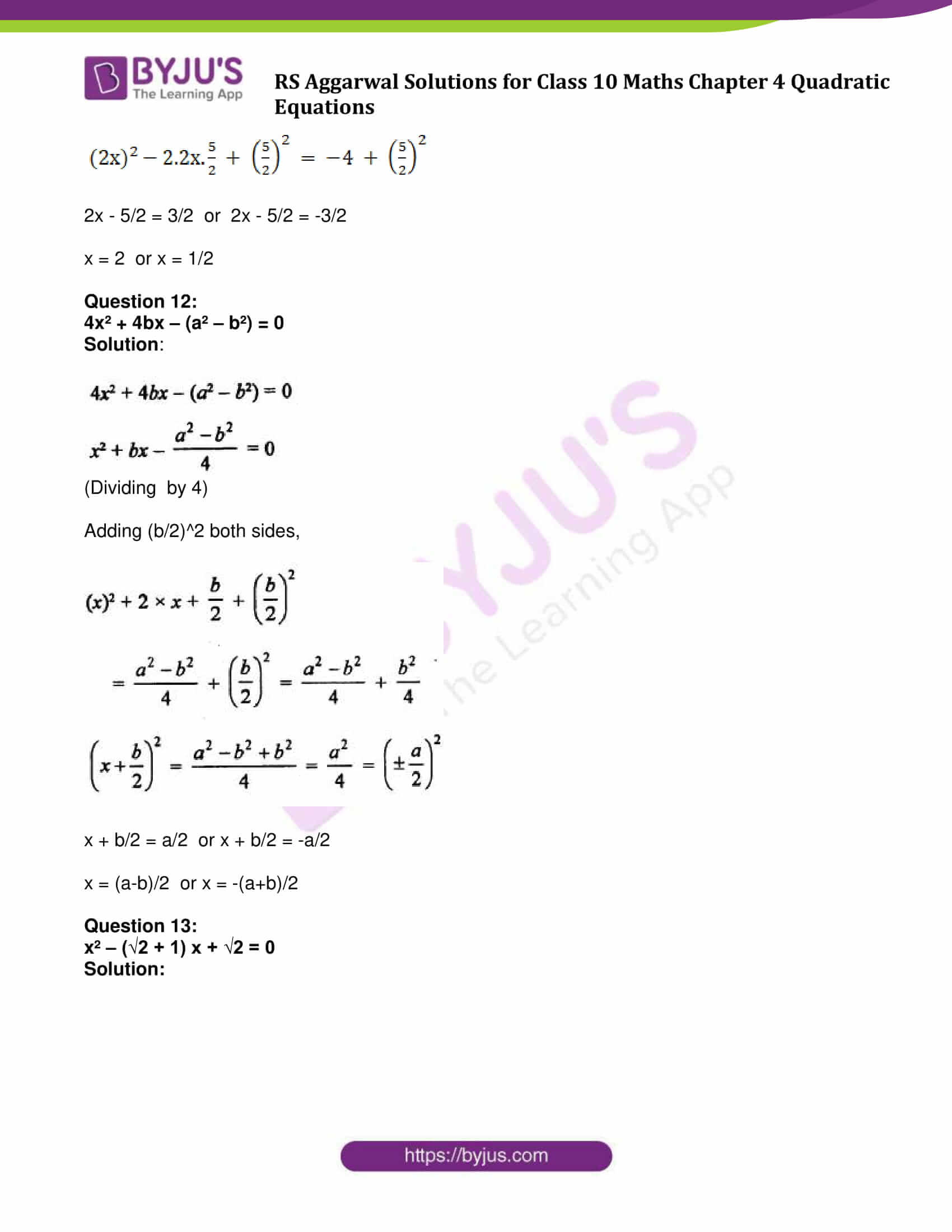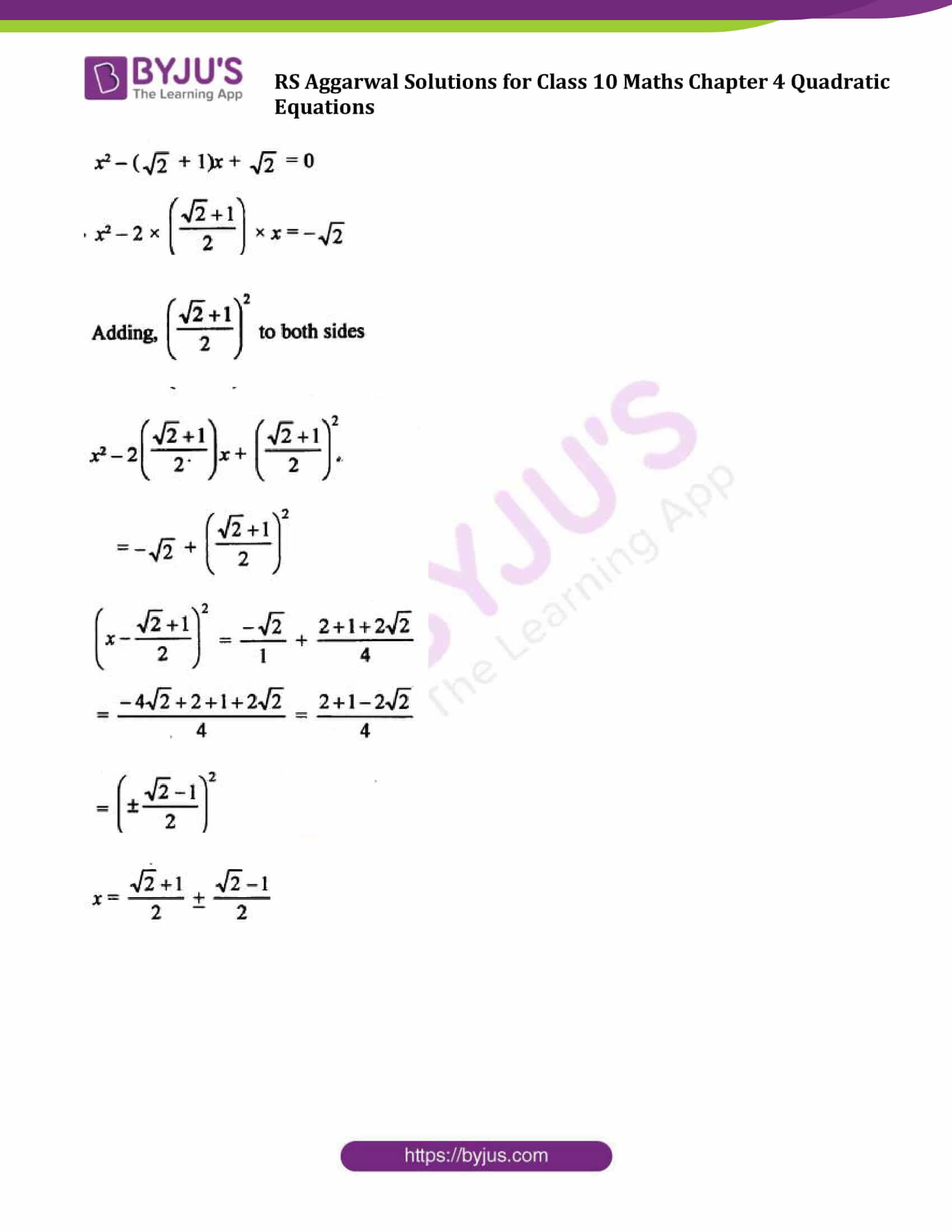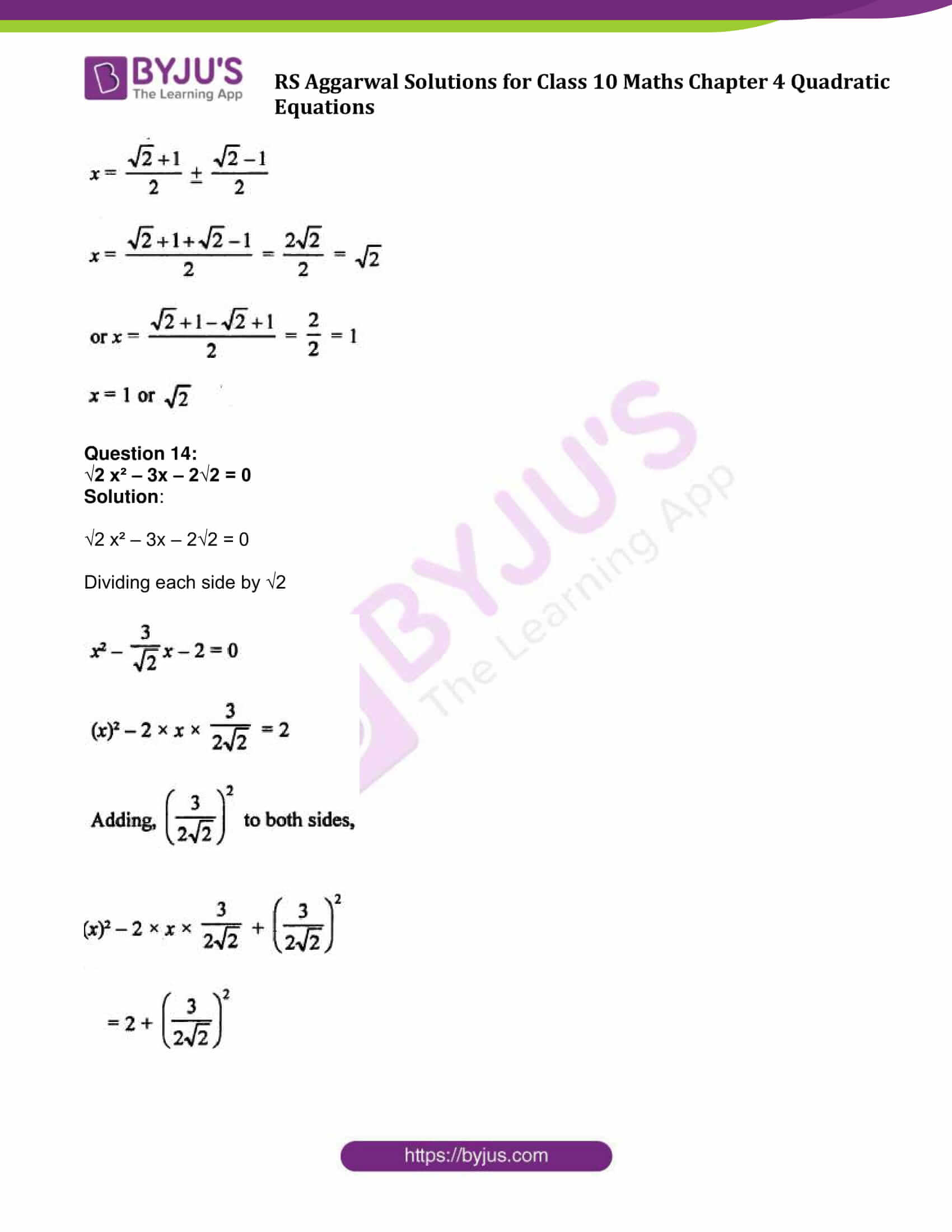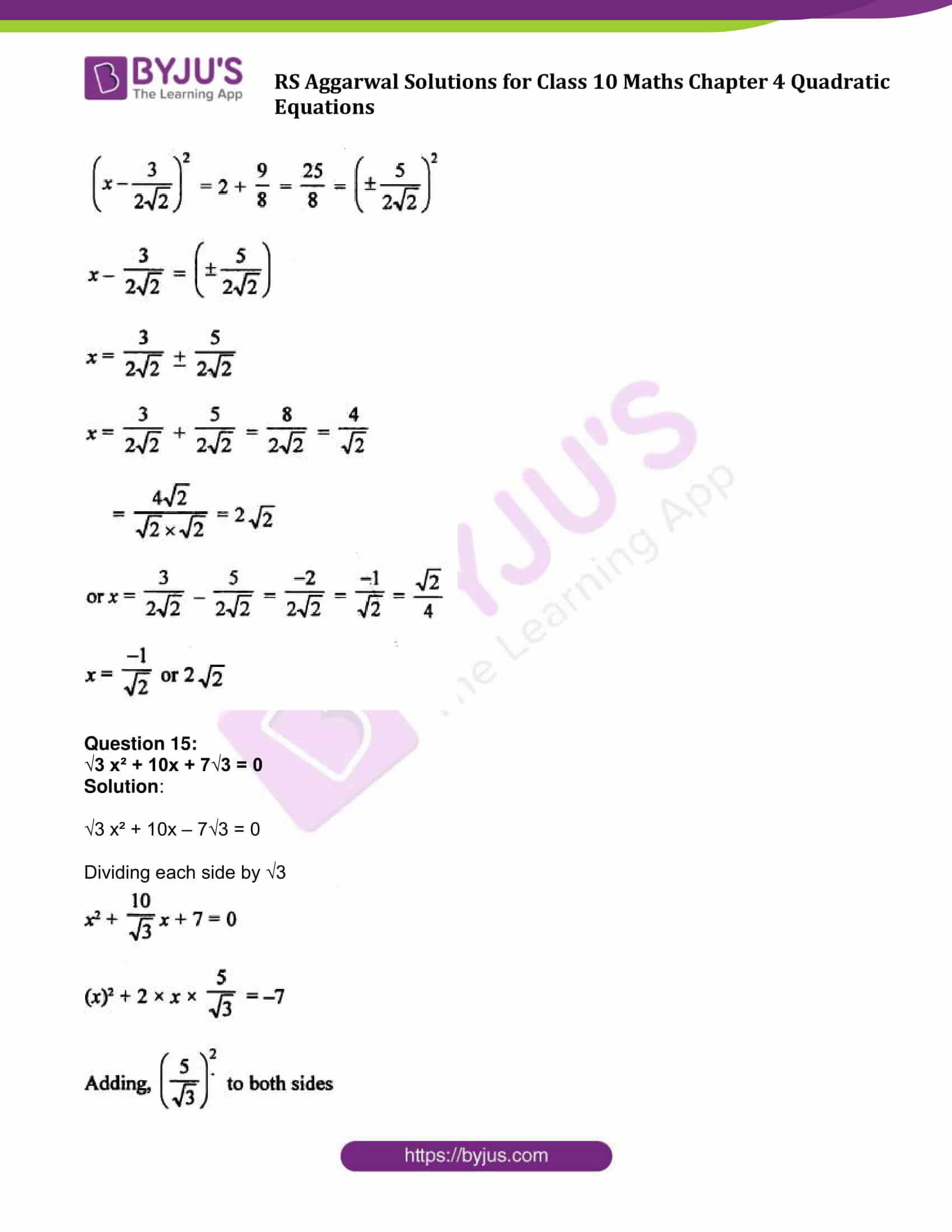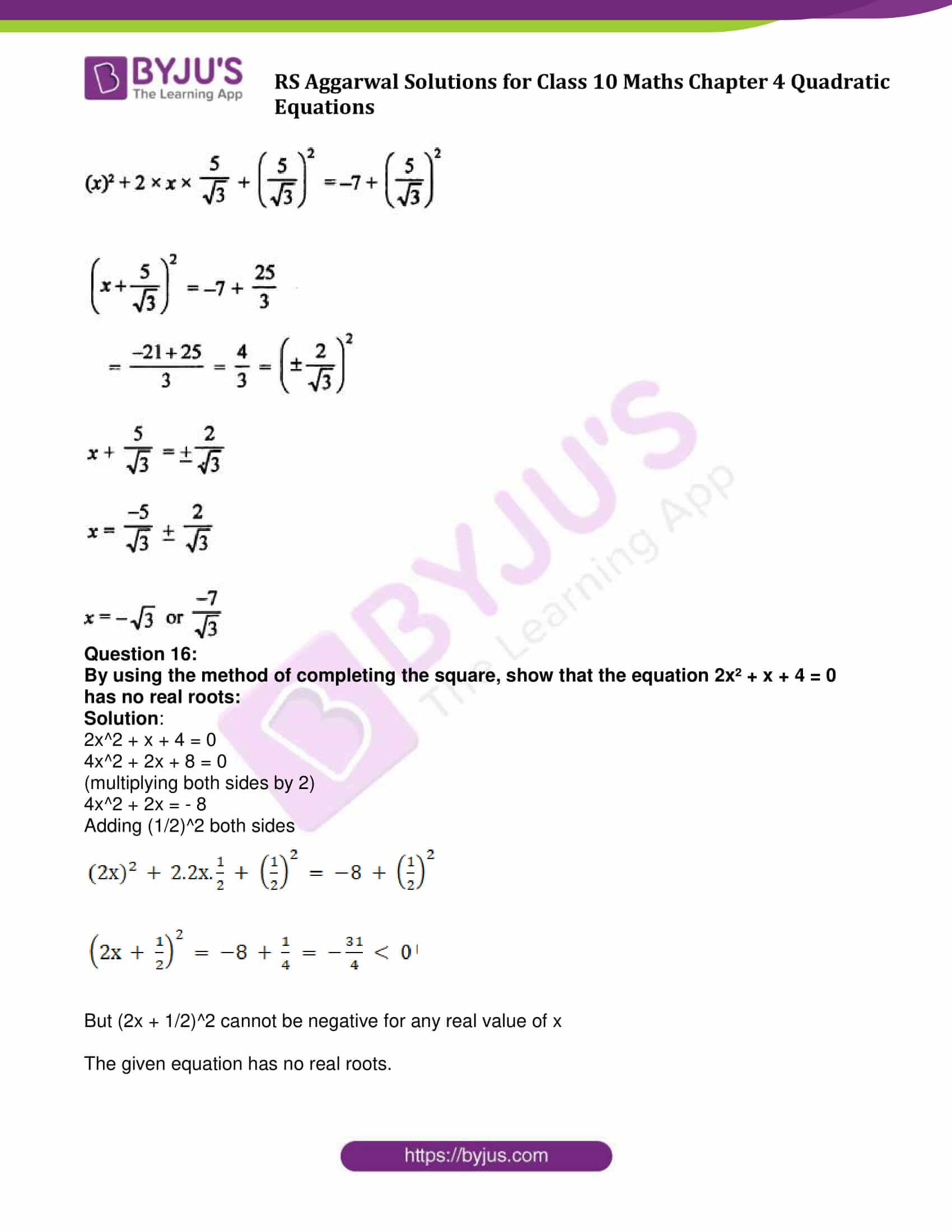### Access other exercise solutions of Class 10 Maths Chapter 4 Quadratic Equations

Exercise 4A Solutions : 30 Questions (Short Answers)

Exercise 4C Solutions : 15 Questions (Short Answers)

Exercise 4D Solutions : 20 Questions (Long Answers)

## Exercise 4B Page No: 185

Solve each of the following equations by using the method of completing the square:

Question 1:

X² – 6x + 3 = 0

Solution:

x² – 6x + 3 = 0

x^2 – 6x + 3 = 0

x^2 – 6x = – 3

x^2 – 2(x)3 + 3^2 = -3 + 3^2

(x – 3)^2 = – 3 + 9 = 6

Using algebraic identity: a^2 – 2ab + b^2 = (a – b)^2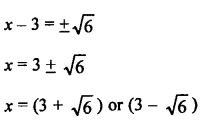Question 2:

x² – 4x + 1 = 0

Solution:

x^2 – 4x + 1 = 0

x^2 – 4x = – 1

x^2 – 2(x)(2) + 2^2 = – 1 + 2^2

(x – 2)^2 = 3

Using algebraic identity: a^2 – 2ab + b^2 = (a – b)^2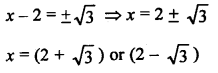Question 3:

x² + 8x – 2 = 0

Solution:

x^2 + 8x – 2 = 0

x^2 + 8x = 2

x2 – 2.x.4 + 42 = 2 + 42

(x + 4)^2 = 18

Using algebraic identity: a^2 – 2ab + b^2 = (a – b)^2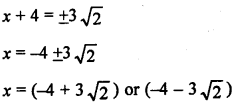Question 4:

4x² + 4√3 x + 3 = 0

Solution: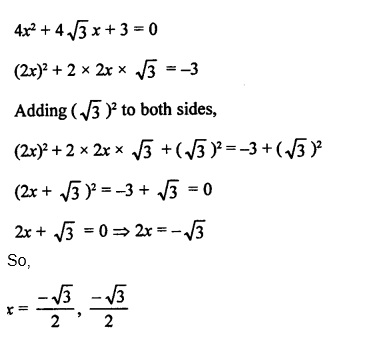Question 5:

2x² + 5x – 3 = 0

Solution:

2x^2 + 5x – 3 = 0

4x^2 + 10x – 6 = 0

(multiplying both sides by 2)

4x^2 + 10x = 6

(2x + 5/2)^2 = 6 + 25/4 = 49/4

Using algebraic identity: a^2 – 2ab + b^2 = (a – b)^2

Taking square root,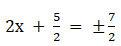2x + 5/2 = 7/2 or 2x + 5/2 = -7/2

x = 1/2 or -3

Question 6:

3x² – x – 2 = 0

Solution:

3x^2 – x – 2 = 0

9x^2 – 3x – 6 = 0

(multiplying both sides by 3)

9x^2 – 3x = 6

Adding (1/2)^2 on both the sides.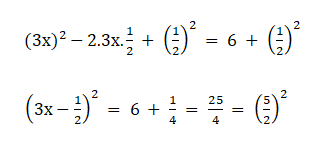3x – 1/2 = 5/2 or 3x – 1/2 = -5/2

x = 1 or x = -2/3

Question 7:

8x² – 14x – 15 = 0

Solution:

8x^2 – 14x – 15 = 0

16x^2 – 28x – 30 = 0

(multiplying both sides by 2)

Adding (7/2)^2 on both the sides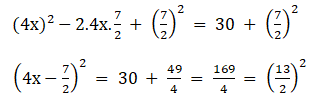4x – 7/2 = 13/2 or 4x – 7/2 = -13/2

x = 5/2 or x = -3/4

Question 8:

7x² + 3x – 4 = 0

Solution:

7x^2 + 3x – 4 = 0

49x^2 + 21x – 28 = 0

(multiplying both sides by 7)

Adding (3/2)^2 on both the sides,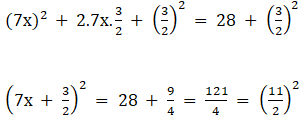7x + 3/2 = 11/2 or 7x + 3/2 = -11/2

x = -1 or x = 4/7

Question 9:

3x² – 2x – 1 = 0

Solution:

3x^2 – 2x – 1 = 0

9x^2 – 6x = 3

(multiplying both sides by 3)

Adding (1)^2 on both the sides

(3x)^2 – 2.3x.1 + (1)^2 = 3 + (1)^2

(3x – 1)^2 = 2^2

3x – 1 = 2 or 3x – 1 = -2

x = -1 or x = -1/3

Question 10:

5x² – 6x – 2 = 0

Solution:

5x^2 – 6x – 2 = 0

25x^2 – 30x – 10 = 0

(multiplying both sides by 5)

25x^2 – 30x = 10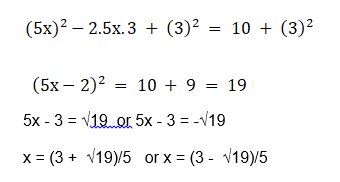Question 11:

2/x^2 – 5/x + 2 = 0

Solution: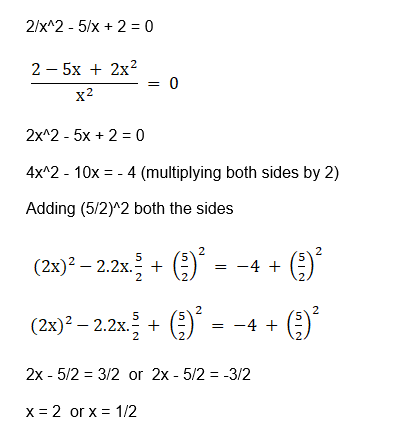Question 12:

4x² + 4bx – (a² – b²) = 0

Solution: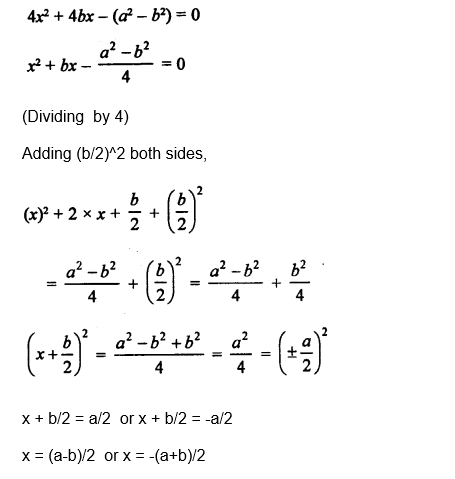Question 13:

x² – (√2 + 1) x + √2 = 0

Solution: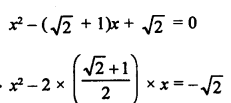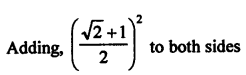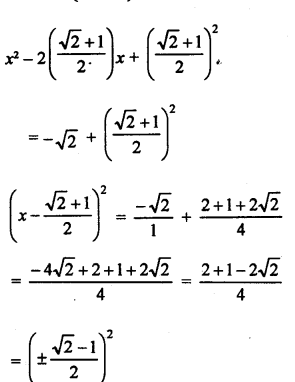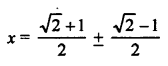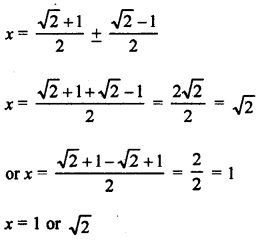Question 14:

√2 x² – 3x – 2√2 = 0

Solution:

√2 x² – 3x – 2√2 = 0

Dividing each side by √2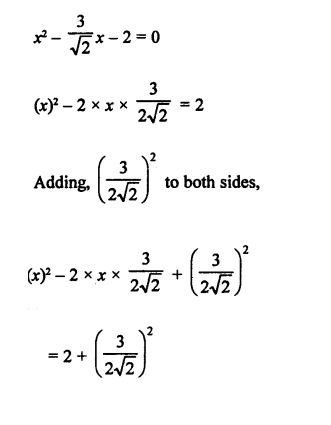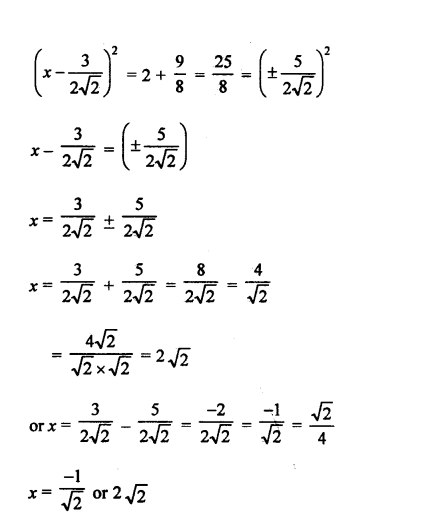Question 15:

√3 x² + 10x + 7√3 = 0

Solution:

√3 x² + 10x – 7√3 = 0

Dividing each side by √3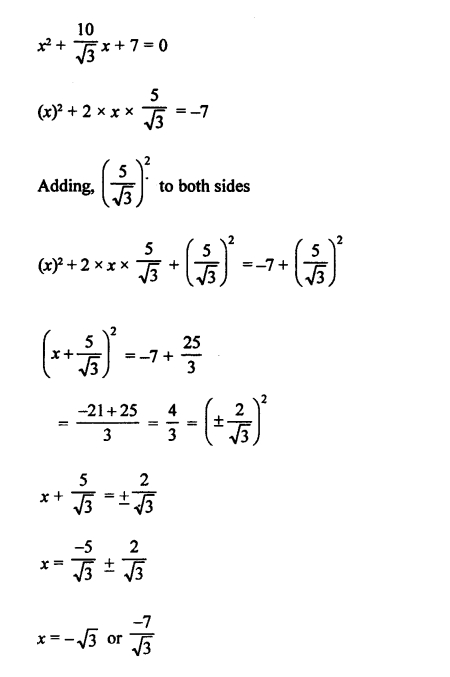Question 16:

By using the method of completing the square, show that the equation 2x² + x + 4 = 0 has no real roots:

Solution: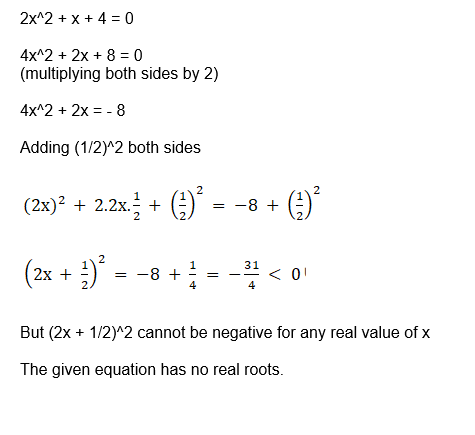## R S Aggarwal Solutions for Class 10 Maths Chapter 4 Quadratic Equations Exercise 4B

Class 10 Maths Chapter 4 Quadratic Equations Exercise 4B is based on the topic, Solving quadratic equations by completing the square method. Download and practice R S Aggarwal Solutions for Class 10 Chapter 4 Quadratic Equations and master the concepts.